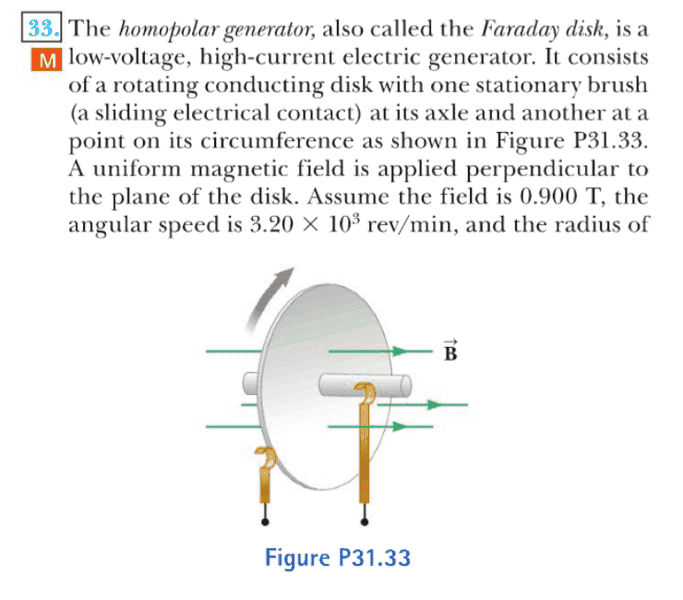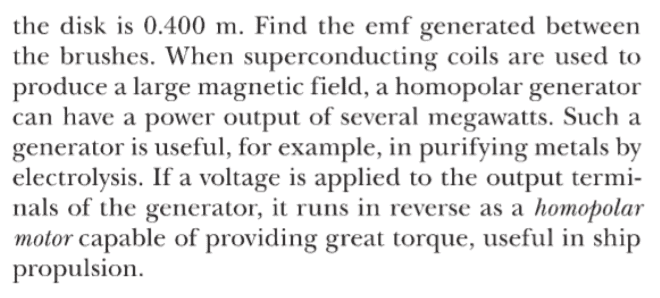# Calculating a Homopolar Generator's EMF

• ElPimiento

## Homework Statement## Homework Equations

##\omega = v/r##
##q\vec v \times \vec B\ =\ \vec F_B##
##|\vec F|d\ =\ |\vec E|##
##q \Delta V\ =\ E##

## The Attempt at a Solution

(So, my answer is twice and large as the correct answer. This is the second time this has happened on a question like this . . .)
Since a negative charge would collect on the outside of the disk I began by analyzing one negative charge and treated it in equilibrium on the edge of the disk,
\begin{align} q\vec v \times \vec B- \frac{|\vec E|}{d}\ &=\ 0 \\ qvB \ &=\ \frac{q \Delta V}{r} \\ vBr \ &=\ \Delta V \end{align}
And since ##v\ =\ \omega r##:
\begin{align} (\omega r)Br \ &=\ \Delta V \\ \Delta V \ =\ \epsilon \ &\approx\ 48.3\ V \end{align}
Any help would be wonderful c:

d(emf) = Bv(r)dr
and show off your powers of integration! :-)

•ElPimiento
I'm back tracking the work a little to make sure i understand where your getting that form of emf:
##\begin{align}
F_B - F_E \ &=\ 0 \\
F_B \ &=\ F_E\ =\ q|\vec E|\ =\ q\frac{d(\epsilon)}{dr} \\
vB\ =\ \omega r B\ &=\ \frac{d(emf)}{dr} \\
\int (\omega r B)dr\ &=\ \epsilon \\
\frac{\omega B r^2}{2} \ &=\ \epsilon\ \approx 24.1\ V
\end{align}##
thanks so much!

I'm back tracking the work a little to make sure i understand where your getting that form of emf:
##\begin{align}
F_B - F_E \ &=\ 0 \\
F_B \ &=\ F_E\ =\ q|\vec E|\ =\ q\frac{d(\epsilon)}{dr} \\
vB\ =\ \omega r B\ &=\ \frac{d(emf)}{dr} \\
\int (\omega r B)dr\ &=\ \epsilon \\
\frac{\omega B r^2}{2} \ &=\ \epsilon\ \approx 24.1\ V
\end{align}##
thanks so much!
Looking good!

d(emf) = B(r) v(r) dr is just the generalized (differential) form of emf = Bvr. Just basic calculus. It allows B and/or v to be functions of r. If B and v are not functions of r then it's just Bvr. But in your case B = constant but v = v(r) so then
d(emf) = B v(r) dr = B ωr dr
and when integrated over r=0 to r= R you get emf = ωBR2/2
where R is the disk's radius.

Last edited: